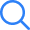# NCERT Solutions For Class 9 Maths

NCERT Solutions for Class 9 Maths comprises the solutions to all the questions given in the NCERT textbook of Class 9. The students can download solutions in PDF for every single chapter, the links provided further below on this page are the direct links to download solutions.

These Class 9 Maths NCERT Solutions cover all the topics given in the NCERT textbook, for instance, Number systems, Coordinate Geometry, Polynomials, Euclid’s Geometry, Quadrilaterals, Triangles, Circles, Constructions, Surface Areas and Volumes, Statistics, Probability, and many more.

With the help of these Solutions of NCERT Books for Class 9 Maths, students can practice the questions from each chapter.

The CBSE Class 9 Maths Solutions have been designed by our subject-matter experts in a well-structured format, to give students several possible methods of answering the problems, and to ensure a proper understanding of concepts given in the book.

The students are advised to practice all these Class 9 Maths NCERT Solutions thoroughly for their classroom test or final exams. It will also help them to build a stronger basic knowledge for the higher classes.

## NCERT Solutions for Class 9 Maths Chapter Wise

In class 9th NCERT Maths Textbook, there are a total of 15 chapters, and having a thorough understanding of all those chapters’ questions is imperative.

Therefore, these chapter-wise NCERT Solutions for Class 9 Maths PDF are provided to help students to grasp in-depth understanding and improve their knowledge to gain more scores in the examinations.

 NCERT Solutions for Class 9 Maths Chapter Wise Chapter 1 Number System Chapter 2 Polynomials Chapter 3 Coordinate Geometry Chapter 4 Linear Equations in Two Variables Chapter 5 Introduction to Euclids Geometry Chapter 6 Lines and Angles Chapter 7 Triangles Chapter 8 Quadrilaterals Chapter 9 Areas of Parallelograms and Triangles Chapter 10 Circles Chapter 11 Constructions Chapter 12 Heron’s Formula Chapter 13 Surface Areas and Volumes Chapter 14 Statistics Chapter 15 Probability

The above-given Chapter wise PDF of NCERT Solutions For Class 9 Maths is accessible to everyone and can be downloaded without login. In addition, it is provided here for Free.

### NCERT Solutions for Class 9 Maths Chapter Wise Exercise

Class 9 Maths NCERT Solutions Chapter Wise Exercises are provided here for the convenience of students. Whether they want to increase their overall marks or a better understanding of all the chapters and concepts, these chapter-wise exercises solutions are adequate. The links to download exercise-wise Class 9 Maths NCERT Solutions are mentioned below.

### NCERT Solutions For Class 9 Maths Chapter 1 Number System - Term 1

Class 9 Maths NCERT Solutions Chapter 1 Number System includes various topics, such as rational numbers and irrational numbers. Students will also be able to extend their knowledge of the number line and how to represent numbers on it. This chapter contains a total of 6 exercises and a good practice in all these exercises can increase the knowledge of the Number Systems to the next level. The chapter also explains the laws of integral powers and rational exponents.

### NCERT Solutions For Class 9 Maths Chapter 2 Polynomials - Term 2

Class 9 Maths Chapter 2 assists students to know about a particular type of algebraic expression called polynomial and the terminology related to it.

In addition to that, it also guides students to learn Remainder Theorem and the Factor Theorem. In this chapter, students will learn various types of definitions of different terms like polynomial, degrees, coefficient, zeros, and terms of a polynomial.

It consists of a total of 5 exercises that aid in a deep understanding of this chapter and its topics. Students preparing for their class 9th maths annual exam can refer to the NCERT Solutions For Class 9 Maths PDF. It will enable them to revise the various types of questions from chapter 2.

### NCERT Solutions For Class 9 Maths Chapter 3 Coordinate Geometry – Term 1

With a total of 3 exercises, this chapter gives students a depth understanding of the Coordinate Geometry. It includes concepts of the cartesian plane, coordinates of a point in XY – plane, terms, and notations associated with the coordinate plane, including the x-axis, y-axis, x- coordinate, y-coordinate, origin, quadrants, etc.

The questions are given in this chapter revolve around the given concepts of coordinate geometry. Browse the below-given links to access the free NCERT Solutions For Class 9 Maths PDF of Coordinate Geometry.

### NCERT Solutions For Class 9 Maths Chapter 4 Linear Equations in Two Variables – Term 1

A total of 4 Exercises are given in this chapter that assists students to recall the knowledge of linear equations in two variables. Along with that, it will also guide the students to learn how to plot the graph of a linear equation in two variables.

A thorough practice of the given chapter will help them understand it better and score more marks. Links to download Class 9 Maths NCERT Solutions Chapter 4 is given below.

### NCERT Solutions For Class 9 Maths 5 Introduction to Euclid's Geometry

There are a total of 2 exercises in Class 9 Maths Chapter 5 Introduction to Euclid’s Geometry. The chapter gives students a detailed introduction to Euclid's approach to geometry and tries to relate it with present-day geometry.

It assists students to learn the methods of defining common geometrical shapes and terms. Practicing the questions given in this chapter will help students to understand it better, therefore links to the questions and their NCERT Solutions For Class 9 Maths PDFs are given in the table here.

### NCERT Solutions For Class 9 Maths Chapter 6 Lines and Angles – Term 1

This chapter includes mostly the theorems that may be asked in the examination to prove. There are many theorems present in this chapter of Lines and Angles and comprehensive knowledge of all those theorems is imperative.

This chapter is not limited to mathematics, but a good knowledge of given topics will help students in science subjects as well. Therefore, good practice in this chapter is required to understand the given topics better. The given NCERT Solutions For Class 9 Maths PDF will help in doing so.

### NCERT Solutions For Class 9 Maths Chapter 7 Triangles – Term 1

This chapter combines various theorems and a detailed explanation of the congruence of triangles, rules of congruence, some properties of triangles, and the inequalities in triangles. It consists of a total of 5 exercises that asks students to prove the properties that they have learned earlier as well. The solution of this chapter will help them to answer those questions in a stepwise manner.

### NCERT Solutions For Class 9 Maths Chapter 8 Quadrilaterals – Term 2

The Quadrilaterals chapter 8 of class 9 maths gives students a depth knowledge of this topic. A total of 2 exercises are present in this chapter that helps students to practice the questions of Quadrilaterals. To answer the asked questions they need to be thorough with a total of the 9 theorems given in this chapter. In addition to that, a detailed and stepwise answer can aid students to boost their learning process and answer the questions more easily. Therefore, the solutions of Quadrilaterals are given here for free of cost.

### NCERT Solutions For Class 9 Maths Chapter 9 Areas of Parallelograms and Triangles

Areas of Parallelograms and Triangles chapter 9 contains 4 exercises in which questions are asked on the basis of given theorems in this chapter. It also guides students to gain knowledge about the formula to find the areas of different figures. The questions in the exercise are mostly based on the proofs.

### NCERT Solutions For Class 9 Maths Chapter 10 Circles – Term 2

Chapter 10 Circles has many theorems, about 12 theorems are given in this chapter and these should be well versed to the students so that they can understand the chapter in-depth and be able to solve the questions. The given six exercises in this chapter ensure students have learned things appropriately and in detail. Links to download the PDF are given here in the table.

### NCERT Solutions For Class 9 Maths Chapter 11 Constructions – Term 2

This chapter gives students the ability to make constructions such as constructions of triangles along with their base, the difference or sum of the remaining two sides, and one base angle with base angle and parameters. This chapter includes a total of 2 exercises. The answers to those exercises are given here in the table that will help students to construct the figures more accurately.

### NCERT Solutions For Class 9 Maths Chapter 12 Heron’s Formula – Term 1

This chapter is very helpful as it helps students to learn how to calculate the area of a triangle if sides are given. This topic is also applicable to calculating areas of quadrilaterals and other polygons by dividing them into triangles. The two given exercises give students the ability to solve the questions based on the heron’s formula. Solutions to this chapter are given in the table here.

### NCERT Solutions For Class 9 Maths Chapter 13 Surface Areas and Volumes – Term 2

Class 9 Maths Chapter 13 Surface Areas and Volumes is an extended version of chapter mensuration that students have studied in earlier classes. This chapter deals with the volume of cubes, cylinders, cuboids, cones, hemispheres, and spheres in terms of their areas and volumes. It has a total of 8 exercises and the questions are based on the given topics and concepts of Surface Areas and Volumes.

### NCERT Solutions For Class 9 Maths Chapter 14 Statistics – Term 1

This chapter helps students learn to represent data in tabular, and graphical drawings. To represent data in a graphical way different graphs such as Bar graphs, Histograms, Frequency polygons, etc. are being used. It also gives students a brief on topics like mean, median, and mode and finds the central tendency with the raw data.

In order to develop a deeper understanding of statistics, the NCERT solutions for class 9 maths in Hindi medium pdf or English medium solutions are the best.

### NCERT Solutions For Class 9 Maths Chapter 15 Probability – Term 2

Probability is the chapter that helps students to learn about the possibilities of an event whether it will happen or not. This chapter is based on real-life incidents so it makes students interested in answering the questions given in the exercise.

There is only one exercise in Probability class 9 maths. In case students face any trouble in solving the problems given in this chapter, they can seek for given solutions here to solve that question. The given table contains a link to download the Class 9 Maths NCERT Solutions Chapter 15 Probability.

This page is created with the intention to provide students with Class 9 Maths NCERT Solutions. Whenever students are stuck with their class 9 maths problems, they can reach this website to download the NCERT Solutions for class 9 maths in Hindi medium & English medium for free.

#### NCERT Solutions Class 9 Maths Chapter 15 Probability Exercises

This page is created with the intention to provide students with Class 9 Maths NCERT solutions. Whenever students are stuck at their class 9 maths problems they can reach this website to download the solutions for free.

### Chapter Wise Study Tips For Class 9 Maths

Students asking “How to Prepare for Class 9 Maths all Chapters” can refer to this page to get Chapter Wise Study Tips For Class 9 Maths.

We have broken the study tips in chapter wise format, where you will find the brief of the lesson, their important formulas, important questions (Make sure you solve them), and finally the study tips to prepare well for the exam.

Number systems (Chapter 1)

As per the definition, the Number system is a way of expressing numbers with the help of digits and symbols in a consistent manner. There are 4 different types of number system:

1. Decimal number system (Base- 10)
2. Binary number system (Base- 2)
3. Octal number system (Base-8)
4. Hexadecimal number system (Base- 16)

Chapter 1 Number system also teaches students the representation of terminating/non-terminating recurring decimals. A good example of recurring decimals isApart from these, this chapter also covers the representation of square roots of 2, 3 and other non-rational numbers on the number line.

#### Number System Chapter 1 Exercises

Ex. 1.1 – 4 Questions (4 short answers)

Ex. 1.2 – 4 Questions (4 short answers)

Ex. 1.3 – 9 Questions (8 short answers, 1 long answer)

Ex. 1.4 – 2 Questions (2 long answers)

Ex. 1.5 – 5 Questions (4 short answers, 1 long answer)

Ex. 1.6 – 3 Questions (3 short answers)

Important Questions of Class 9 Number System

 Important Questions of Number System Q.1 Find five rational numbers between 1 and 2. Q.2 Locate √3 on the number line. Q.3 Find the decimal expansions of 10/3, 7/8 and 1/7 Q.4 Visualise 3.765 on the number line, using successive magnification. Q.5 Rationalise the denominator of 1/[7+3√3]. Q.6 What is the value of (256)0.16 X (256)0.09?

Important Formula of Class 9 Number System

Number system has many laws and formulas and the knowledge of it helps students to answer the questions with less efforts.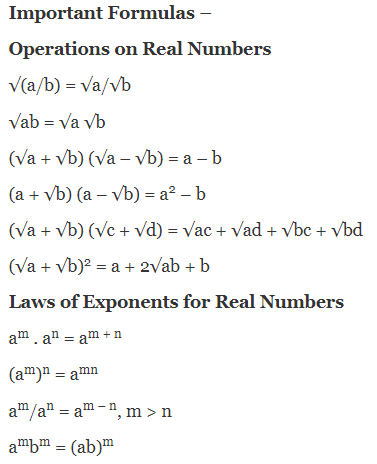Study Tips for Class 9 Number System

Study Tip for number systems is to pay attention to each type of number system one-by-one. Since, it is a core of higher mathematics terms, master all the given number systems.

The image given here represents the number system chart. Learn them properly to be able to answer all the complex or easy answers of Real Numbers.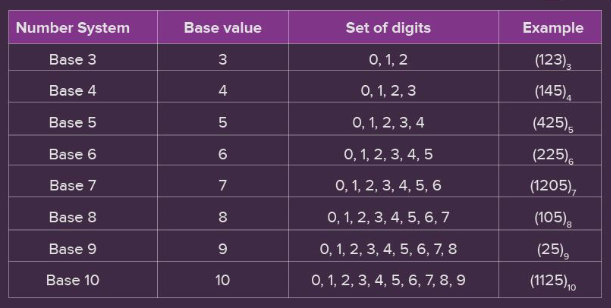Polynomials (Chapter 2)

In class 9th Maths Polynomials covers the following topics:

1. Polynomials in One Variable
2. Zeros of a Polynomial
3. Remainder Theorem
4. Factorization of Polynomials
5. Algebraic Identities

Some important points to remember in polynomials are: polynomials of degree 1 are called linear, degree 2 is called quadratic, degree 3 is called cubic.

Polynomials involve the mathematical operations of addition, subtraction, multiplication, and non-negative integer exponents of variables.

This lesson is very important to cover as it creates the basics stronger of algebra.

#### Polynomials Chapter 2 Exercises

Ex. 2.1 – 5 Questions (5 short answers)

Ex. 2.2 – 4 Questions (4 short answers)

Ex. 2.3 – 3 Questions (3 short answers)

Ex. 2.4 – 5 Questions (4 short answers, 1 long answer)

Ex. 2.5 – 16 Questions (9 short answers, 5 long answers, 2 very long answers)

Important Questions of Class 9 Polynomials

 Important Questions of Polynomials Q.1 Find the value of the polynomial 5x – 4x2 + 3 at x = 2 and x = –1. Q.2 Calculate the perimeter of a rectangle whose area is 25x2 – 35x + 12. Q.3 If (x – 1/x) = 4, then evaluate (x2 + 1/x2) and (x4 + 1/x4). Q.4 Check whether (7 + 3x) is a factor of (3x3 + 7x). Q.5 Factorise x2 – 1 – 2a – a2.

Important Formula of Class 9 Polynomials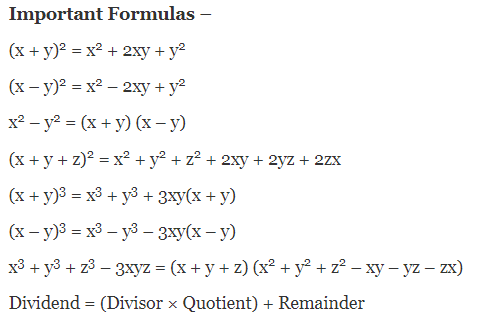Study Tips for Class 9 Polynomials

The study tip for this chapter is to go through all the theorems: Remainder theorem, Factor theorem and solve various questions on these.

A better grip on these will allow you to answer all the questions asked in NCERT Class 9th Maths Chapter 2. Also, learn all the important formulas of Polynomials.

Coordinate Geometry (Chapter 3)

Study of geometry using coordinates is called the Coordinate Geometry. There are several important terms related to the Coordinate Geometry a student should know about. Those terms are as mentioned in the below given image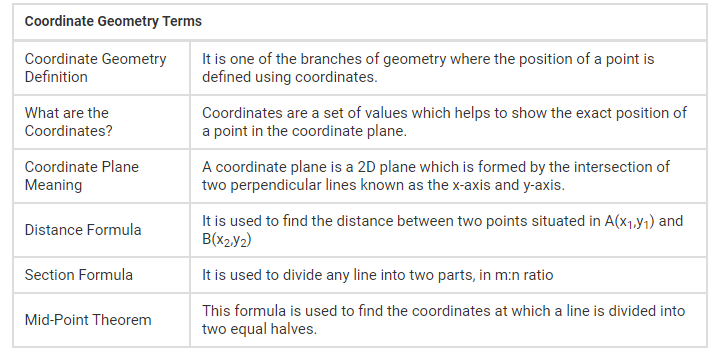In class 9th, Cartesian System and Plotting a Point in the Plane if its Coordinates are Given has been discussed briefly.

Abscissa and ordinates of a point along with that plotting and naming a point in xy – plane has been also covered in this lesson.

#### Coordinate Geometry Chapter 3 Exercises

Ex. 3.1 – 2 Questions (1 short answer, 1 long answer)

Ex. 3.2 – 2 Questions (2 short answers)

Ex. 3.3 – 2 Questions (1 short answer, 1 long answer)

Important Questions of Class 9 Coordinate Geometry

Q.1:- Solve the following equations: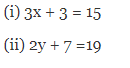Q.2:- Plot the following points and check whether they are collinear or not: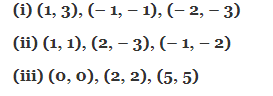👉 Important Formula of Class 9 Coordinate Geometry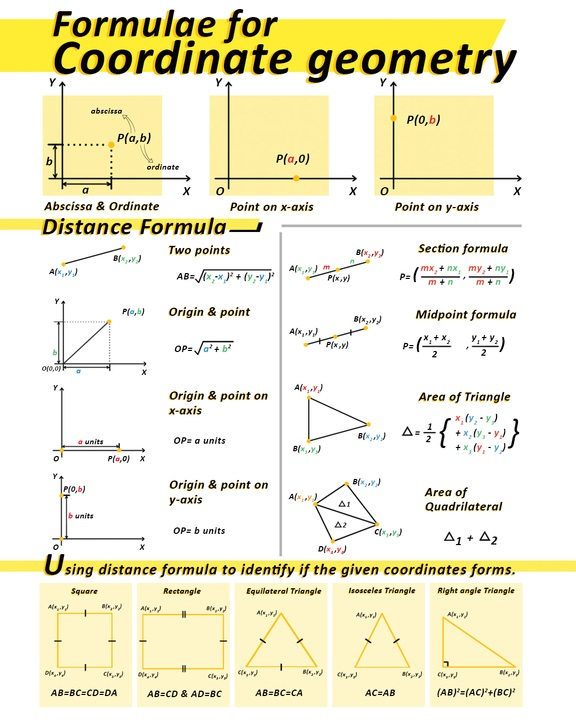In order to understand the above given important formulae of coordinate geometry it is important to understand the terms:-

1. The plane has two names: the Cartesian, or coordinate plane and the lines are called the coordinate axes.
2. The x -axis, lies horizontally where the y - axis lies vertically.
3. The coordinate axes divide the plane into four parts called quadrants.
4. The point of intersection of the axes is called the origin.
5. Abscissa is known as the distance of a point from the y -axis and the y - coordinate or ordinate is known when the distance of a point is from the x-axis.

Study Tips for Class 9 Coordinate Geometry

Preparation tips for Coordinate Geometry is to be thorough with the terms mentioned and learn properly how to plot a point using coordinates.

Remember the definitions of Abscissa, Ordinate, origin, about axis, and more.

Linear Equations in Two Variables (Chapter 4)

About 6 to 7 questions can be asked from the linear equation in two variables chapter 4.

As per the mathematics exam pattern of 9th grade 5 types of questions can be created from this topic.

Those 5 types are MCQs, Very Short Answer Types of Questions, Short Answer Types of Question, Long Answer Types of Questions and Long Short Answer Questions.

Each type of question has different marks and thus, you must pay attention to the NCERT Class 9 Maths Chapter 4 to answer all the questions with ease.

#### Linear Equations in Two Variables Chapter 4 Exercises

Ex. 4.1 – 2 Questions (2 short answers)

Ex. 4.2 – 4 Questions (3 short answers, 1 long answer )

Ex. 4.3 – 8 Questions (4 short answers, 4 long answers)

Ex. 4.4 – 2 Questions (2 long answers)

The study tip for Linear equation of class ninth is to practice a lot of word questions.

Introduction to Euclid Geometry (Chapter 5)

The NCERT 9th grade mathematics chapter 5 Introduction to Euclid’s Geometry is the base of very advanced topics.

Although, for class 9th students they have an opportunity to score 1-2 marks from this lesson as per the CBSE 9th Maths Syllabus.

#### Introduction to Euclid’s Geometry Chapter 5 Exercises

Ex. 5.1 – 7 Questions (4 short answers, 3 long answers)

Ex. 5.2 – 2 Questions (1 short answer, 1 long answer)

Students who want to score good marks from this chapter should focus on the important questions that are often asked in the exam.

Important Questions of Class 9 Introduction to Euclid Geometry

Q:-What are the five postulates of Euclid’s Geometry?

Q:-Does Euclid’s fifth postulate imply the existence of parallel lines? Explain.

Q:-In the given figure, if AC = BD, then prove that AB = CD.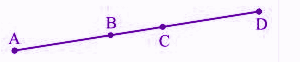You can access the chapter-wise important questions for Class 9 Maths with solutions to practice all the important questions of Euclid’s Geometry.

Go through them and solve the questions a lot to develop a deeper and better understanding in Euclid’s Geometry.

Study Tips for Class 9 Introduction to Euclid Geometry

Euclid’s Definitions of Axioms and Postulates as well as all the 5 Postulates are important to know.

You should have a good knowledge about all the axioms and postulates mentioned in the NCERT Class 9 maths Textbook.

Apart from this, you should be profound in solving the theorems given in the lesson.

Following is the theorem:- Prove that, Two distinct lines cannot have more than one point in common.

Also, the Euclid’s Five Postulates you should know about are:-

Postulate 1 – A straight line may be drawn from any one point to any other point.

Postulate 2 – A terminated line can be produced indefinitely.

Postulate 3 – A circle can be drawn with any centre and any radius.

Postulate 4 – All right angles are equal to one another.

Postulate 5 – If a straight line falling on two straight lines makes the interior angles on the same side of it taken together less than two right angles, then the two straight lines, if produced indefinitely, meet on that side on which the sum of angles is less than two right angles.

Lines and Angles (Chapter 6)

There are 3 Exercises in class 9 Maths Chapter 6 that contain short answer questions and long answer questions. This entire lesson discusses the theorems on Lines and Angles.

Four axioms and eight theorems are available in Chapter 6 of class 9th mathematics that are important to solve.

In the annual examination, often the maths questions ask the differences between line and line segment, hence it is an important topic to cover.

Apart from this, the chapter 6 of 9th std maths contains 4 basic terms:- Point, Line, Line Segment, Ray.

Basic Terms to be covered in Lines and Angles Chapter 6 are:-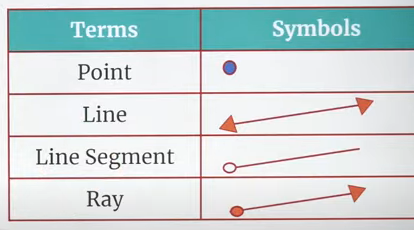Types of Angle

Below given image represent the types of angles, their size of angle and the example how they would look.#### Lines and Angles Chapter 6 Exercises

Ex. 6.1 – 6 Questions (5 short answers, 1 long answer)

Ex. 6.2 – 6 Questions (3 short answers, 3 long answers)

Ex. 6.3 – 6 Questions (5 short answers, 1 long answer)

👉 Important Questions of Class 9 Lines and Angles

Q:- In the figure, if AB || CD || EF, PQ || RS, ∠RQD = 25° and ∠CQP = 60°, then find ∠QRS.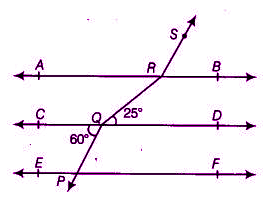Q:- In the figure, lines AB and CD intersect at O. If ∠AOC + ∠BOE = 70° and ∠BOD = 40°, find ∠BOE and reflex ∠COE.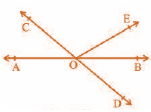Apart from this, there are many important questions, make sure you go through them. To find them refer to the previous year question papers and sample question papers.

Study Tips for Class 9 Lines And Angles

The lines and Angles chapter 6 is an interesting lesson to cover however, solving the questions needs an immense focus and good grip on the basics definition of the given topic.

To prepare for this topic, We would like to suggest you to go through the NCERT Class 9th Maths Textbooks and try to solve the examples.

In addition to that, go through the whole exercises and refer to some additional reference textbooks to practice the questions as much as possible.

Only practicing the questions can help you to master the topic lines and angles.

Triangles (Chapter 7)

Chapter 7 Triangles is an important chapter as it holds 5-7 marks weightage. In this lesson, students will have to learn about triangles, its properties, congruence of triangles, rules of congruence, inequalities in triangles and more.

The chapter contains a total of 5 exercises which ask the several questions on proving the statement given.

The exercises also contain questions that need to be answered by the students based on the previous classes' learning.

There are 8 theorems in this chapter and all of these are important to learn since the questions are asked in the examination based on those theorems.

#### Triangles Chapter 7 Exercises

Ex. 7.1 – 8 Questions (6 short answers, 2 long answers)

Ex. 7.2 – 8 Questions (6 short answers, 2 long answers)

Ex. 7.3 – 5 Questions (3 short answers, 2 long answers)

Ex. 7.4 – 6 Questions (5 short answers, 1 long answer)

Ex. 7.5 – 4 Questions (3 short answers, 1 long answer)

Axioms and Theorems

Axiom 7.1 (SAS congruence rule) – As per the congruence rule of SAS, if two sides and the included angle of one triangle are equal to the two sides and the included angle of the other triangle then it will be called the SAS congruence.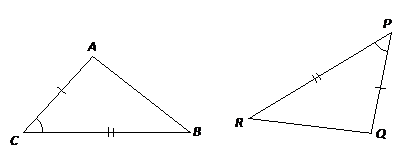Theorem 7.1 (ASA congruence rule) – Angle-Side-Angle congruence rule states: Two triangles are congruent if two angles and the included side of one triangle are equal to two angles and the included side of the other triangle.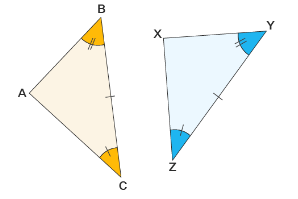Theorem 7.2 – Angles opposite to equal sides of an isosceles triangle are equal.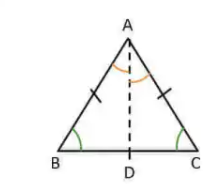Theorem 7.3 – The sides opposite to equal angles of a triangle are equal.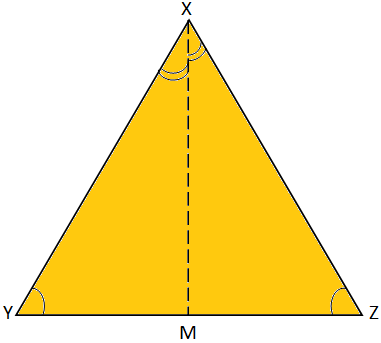Theorem 7.4 (SSS congruence rule) – If three sides of one triangle are equal to the three sides of another triangle, then the two triangles are congruent.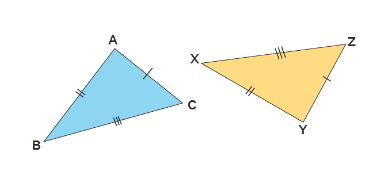Theorem 7.5 (RHS congruence rule) – If in two right triangles the hypotenuse and one side of one triangle are equal to the hypotenuse and one side of the other triangle, then the two triangles are congruent.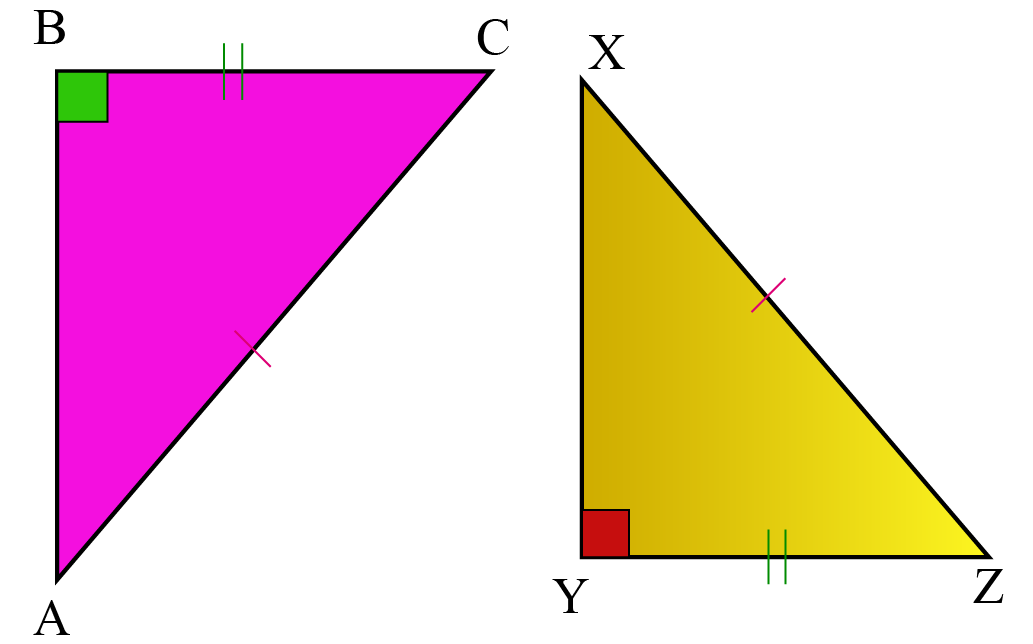Theorem 7.6 – If two sides of a triangle are unequal, the angle opposite to the longer side is larger (or greater).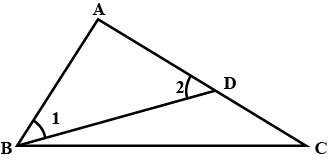Theorem 7.7 – In any triangle, the side opposite to the larger (greater) angle is longer.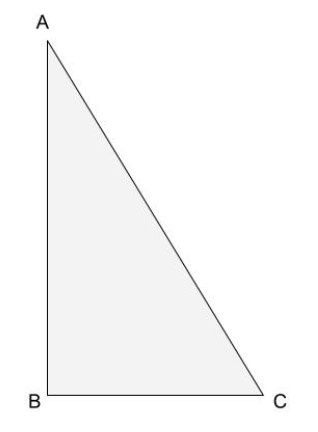Theorem 7.8 – The sum of any two sides of a triangle is greater than the third side.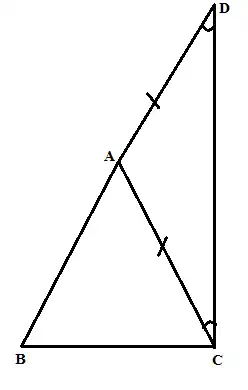Study Tips for Class 9 Triangles

The study tip for chapter 7 triangle is to learn about the triangle, its properties, congruence of triangles and rules of congruence because it holds the 5-7 marks weightage.

You can use the NCERT Class 9 Maths Chapter 7 Triangles Notes PDF to recall the theorems time and again.

The chapter starts with the definition of quadrilaterals: A figure obtained by joining four points in order, with no 3 points collinear. Furthermore, the lesson discusses the types of Quadrilaterals in detail.

There are a total of 1 theorem (mid-point theorem) to prove but questions asked in the exercises can be solved by using the 9 theorems.

All those theorems are available in the NCERT 9th Mathematics book and questions have been asked throughout the given 2 exercises.

#### Quadrilaterals Chapter 8 Exercises

Ex. 8.1 – 12 Questions (2 short answers, 6 long answers, 4 very long answers)

Ex. 8.2 – 7 Questions (2 short answers, 2 long answers, 3 very long answers)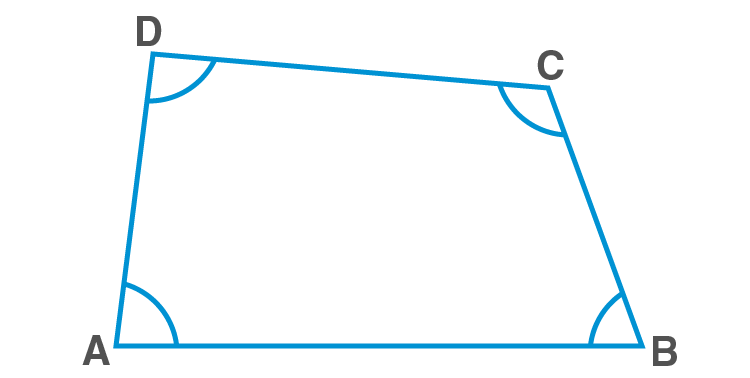A quadrilateral has four sides, four angles and four vertices.

Angle Sum Property of a Quadrilateral – The sum of the angles of a quadrilateral is 360o.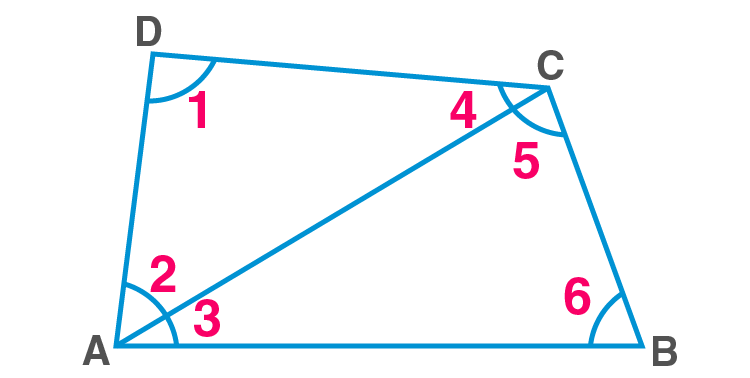Theorem 8.1 – A diagonal of a parallelogram divides it into two congruent triangles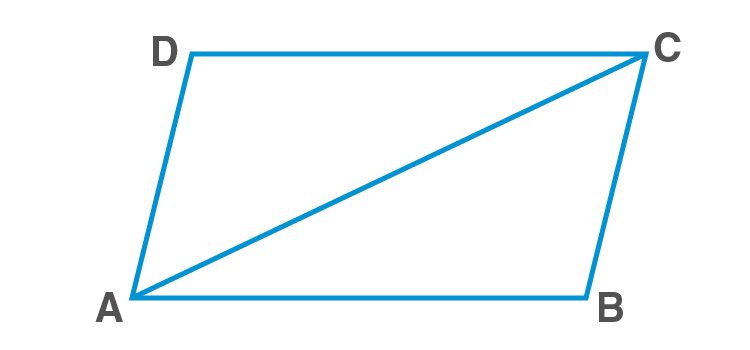Theorem 8.2 – In a parallelogram, opposite sides are equal.Theorem 8.3 – If each pair of opposite sides of a quadrilateral is equal, then it is a parallelogram.

Theorem 8.4 – In a parallelogram, opposite angles are equal.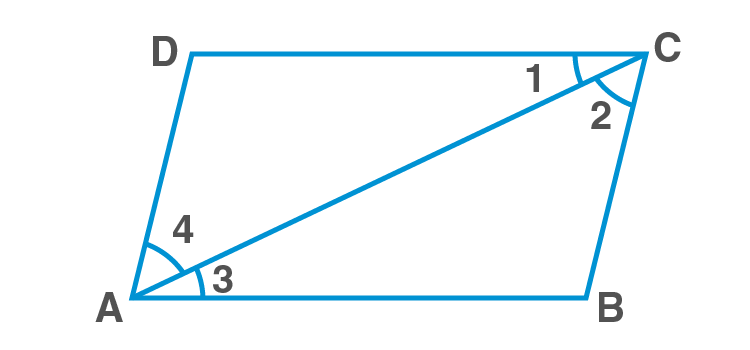Theorem 8.5 – If in a quadrilateral, each pair of opposite angles is equal, then it is a parallelogram.

Theorem 8.6 – The diagonals of a parallelogram bisect each other.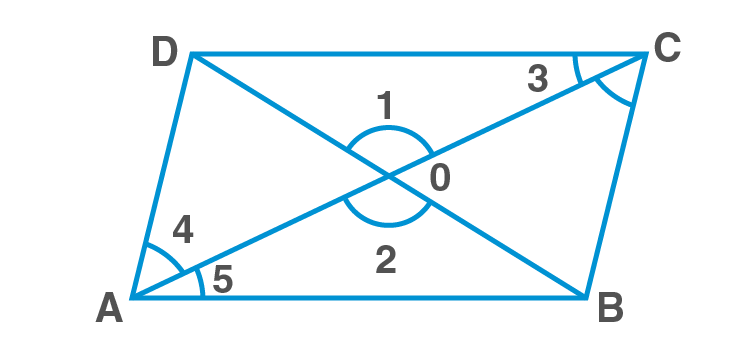Theorem 8.7 – If the diagonals of a quadrilateral bisect each other, then it is a parallelogram.

Theorem 8.8 – A quadrilateral is a parallelogram if a pair of opposite sides is equal and parallel.

Theorem 8.9 – The line segment joining the mid-points of two sides of a triangle is parallel to the third side.

Theorem 8.10 – The line drawn through the mid-point of one side of a triangle, parallel to another side bisects the third side.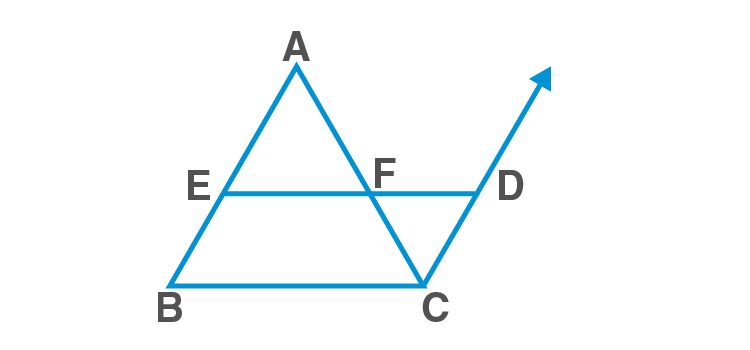👉 Study Tips for Class 9 Quadrilaterals

Go through the Class 9 Quadrilaterals Study Notes and make sure you have a detailed knowledge about the quadrilaterals, their types, properties and the proofs that are given in the lesson.

Note:- Practice the same question time and again so that you can explain to someone how you solved them.

Areas of Parallelograms and Triangles (Chapter 9)

In this lesson, there are 4 exercises and 3 important theorems which must be covered by a 9th standard student. Areas of Parallelograms and Triangles are the study of geometric figures.

In this chapter 9, the formulae and theorems have been explained so that students can learn the ways to find the areas of different figures, by studying relationships between the areas of geometric figures.

#### Areas of Parallelograms and Triangles Chapter 9 Exercises

Ex.9.1 – 1 Question (1 short answer)

Ex. 9.2 – 6 Questions (5 short answers, 1 long answer)

Ex. 9.3 – 16 Questions (12 short answers, 4 long answer)

Ex. 9.4 – 8 Questions (4 short answer, 1 long answer, 3 very long answers)

Important Theorems Areas of Parallelograms and Triangles

Theorem 9.1 – Parallelograms on the same base and between the same parallels are equal in area.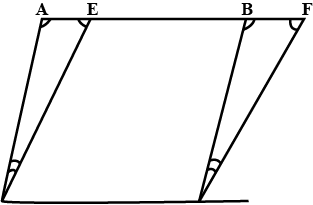Theorem 9.2 – Two triangles on the same base (or equal bases) and between the same parallels are equal in area.Theorem 9.3 – Two triangles having the same base (or equal bases) and equal areas lie between the same parallels.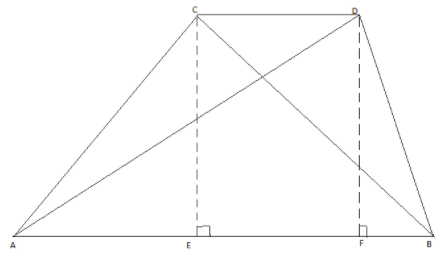Study Tips for Class 9 Areas of Parallelograms and Triangles

A detailed knowledge of all the theorems and given properties of all parallelograms and Triangles are important, so make sure to develop a good understanding in these things.

Additionally, lots of practice to all the questions are recommended.

Circles (Chapter 10)

According to the definition of circle, it is a collection of all the points in a plane, at a fixed distance from a fixed point in the plane. This lesson allows students to cover the topics like Angle Subtended by a Chord at a Point, Equal Chords and their respective distances from the Centre, the Angle Subtended by an Arc of a Circle, etc.

Along with that there are 12 theorems which must be covered by the students to understand the concepts clearly.

Among the given 6 exercises, questions are given from all the essential topics of the circle.

#### Circle Chapter 10 Exercises

Throughout the given six exercises in chapter 10 of NCERT 9th maths, there are short answer types of questions as well as long answer types of questions.

Ex. 10.1 – 2 Questions (2 short answers)

Ex. 10.2 – 2 Questions (2 long answers)

Ex. 10.3 – 3 Questions (3 long answers)

Ex. 10.4 – 6 Questions (6 long answers)

Ex. 10.5 – 12 Questions (12 long answers)

Ex. 10.6 – 10 Questions (10 long answers)

Important Theorems Of Circle

There are 12 theorems of circle all the 9th standard students should study. Below we have mentioned all the twelve circle’s theorems, give them a read.

Theorem 10.1 – Equal chords of a circle subtend equal angles at the centre.

Theorem 10.2 – If the angles subtended by the chords of a circle at the centre are equal, then the chords are equal.

Theorem 10.3 – The perpendicular from the centre of a circle to a chord bisects the chord.

Theorem 10.4 – The line drawn through the centre of a circle to bisect a chord is perpendicular to the chord.

Theorem 10.5 – There is one and only one circle passing through three given non-collinear points.

Theorem 10.6 – Equal chords of a circle (or of congruent circles) are equidistant from the centre (or centres).

Theorem 10.7 – Chords equidistant from the centre of a circle are equal in length.

Theorem 10.8 – The angle subtended by an arc at the centre is double the angle subtended by it at any point on the remaining part of the circle.

Theorem 10.9 – Angles in the same segment of a circle are equal.

Theorem 10.10 – If a line segment joining two points subtends equal angles at two other points lying on the same side of the line containing the line segment, the four points lie on a circle (i.e. they are concyclic).

Theorem 10.11 – The sum of either pair of opposite angles of a cyclic quadrilateral is 180º.

Theorem 10.12 – If the sum of a pair of opposite angles of a quadrilateral is 180º, the quadrilateral is cyclic.

Study Tips for Class 9 Circle

Whatever you will learn in this lesson, will help you across all the classes (if maths is chosen to study).

Since 9th is a beginner level standard so the topics like Circles are very important as it builds the base.

Hence, make sure to spend plenty of time mastering all the given 12 theorems. And considered solving lots of questions from the NCERT Exemplar maths book of class 9th.

Constructions (Chapter 11)

Chapter 11 of NCERT Class 9th Mathematics deals with the basic constructions where students are taught various methods to construct the certain kinds of triangles.

There are two exercises that deal with several questions. The first exercise contains the questions that are based on constructing the angles whilst the second exercise deals with constructions of triangles, when different parameters are given.

#### Constructions Chapter 11 Exercises

Ex. 11.1 – 5 Questions (2 short answers, 2 long answers, 1 very long answer)

Ex. 11.2 – 5 Questions (5 very long answer)

Important Constructions To Learn

The NCERT 9th standard mathematics textbook contains 6 important constructions/points, a student must learn them properly.

Construction 11.1 – To construct the bisector of a given angle.

Construction 11.2 – To construct the perpendicular bisector of a given line segment.

Construction 11.3 – To construct an angle of 600 at the initial point of a given ray.

Construction 11.4 – To construct a triangle, given its base, a base angle and sum of other two sides.

Construction 11.5 – To construct a triangle given its base, a base angle and the difference of the other two sides.

Construction 11.6 – To construct a triangle, given its perimeter and its two base angles.

Study Tips for Class 9 Constructions

The construction is considered as one of the easiest topics to understand as well as to apply. A student can score 3-4 marks from this topic so, it is important to learn all the important Constructions. To study them properly, it is advised to go through all the examples available in the book.

Heron’s Formula (Chapter 12)

When all the three lengths of triangle are given, the Heron’s Formula is used to find the area of a triangle. It is one of the most famous formulas in mathematics especially, among the 9th standard students.

Heron's formula is not only helpful to find the area of a triangle instead, it is useful to calculate the areas of quadrilaterals and other polygons by dividing them into triangles.

In 9th class mathematics, there are two exercises to practice the questions based on Heron’s formula.

#### Constructions Chapter 12 Exercises

Ex. 12.1 – 6 Questions (2 short answers, 2 long answers, 2 very long answers)

Ex. 12.2 – 9 Questions (4 long answers, 5 very long answers)

Important Formula of Heron’s Formula

Area of a triangle = 1/2 × base × height

Area of a triangle by Heron’s Formula =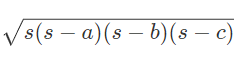Where a, b and c are the sides of a triangle, s is the semi perimeter.

Surface Areas and Volumes (Chapter 13)

The chapter 13 Surface Areas and Volumes is just an extended version of mensuration that students have studied earlier.

However, Apart from the cuboids and cylinders, this lesson surface areas and volumes has broadened the study to some solids such as cones and spheres.

There are 8 exercises in this chapter that will help students to clear their doubts and build the basics knowledge stronger.

#### Surface Areas and Volumes Chapter 13 Exercises

Ex. 13.1 – 8 questions

Ex. 13.2 – 11 questions

Ex. 13.3 – 8 questions

Ex. 13.4 – 9 questions

Ex. 13.5 – 9 questions

Ex. 13.6 – 8 questions

Ex. 13.7 – 9 questions

Ex. 13.8 – 10 questions

Ex. 13.9 – 3 questions

Important Formula of Surface Areas and Volumes Chapter 13

Surface Area of a Cuboid = 2(lb + bh + hl)

where l, b and h are respectively the three edges of the cuboid

Surface Area of a Cube = 6a2

where a is the edge of the cube.

Curved Surface Area of a Cylinder = 2πrh

where r is the radius of the base of the cylinder and h is the height of the cylinder

Total Surface Area of a Cylinder = 2πr(r + h)

where h is the height of the cylinder and r its radius

Curved Surface Area of a Cone = 1/2 × l × 2πr = πrl

where r is its base radius and l its slant height

Total Surface Area of a Cone = πrl + πr2 = πr(l + r)

Surface Area of a Sphere = 4 π r2

where r is the radius of the sphere.

Curved Surface Area of a Hemisphere = 2πr2

where r is the radius of the sphere of which the hemisphere is a part.

Total Surface Area of a Hemisphere = 3πr2

Volume of a Cuboid = base area × height = length × breadth × height

Volume of a Cube = edge × edge × edge = a3

Volume of a Cylinder = πr2h

where r is the base radius and h is the height of the cylinder.

Volume of a Cone = 1/3 πr2h

where r is the base radius and h is the height of the cone.

Volume of a Sphere = 4/3 πr3

where r is the radius of the sphere.

Volume of a Hemisphere = 2/3 πr3

where r is the radius of the hemisphere.

Study Tips for Class 9 Surface Areas and Volumes

Formulae play an important role in this type of chapter so, it is advised to learn/remember all the Surface Areas and Volumes and revise them from time to time.

Apart from this tip, you are suggested to solve a lot of questions to become fluent in solving the questions.

Statistics (Chapter 14)

Data has become an inseparable part of life and so it is important to learn how to manage them. The chapter 14 statistics is the branch of mathematics that helps students to learn how to represent and manage the data/numbers in a readable format.

The chapter helps students to learn how to graphically represent the data, using different graphs such as Bar graphs, Histograms, Frequency polygons, etc.

Also, calculating the mean, median and mode of the raw data has been covered in this lesson.

#### Statistics Chapter 14 Exercises

There are a total of 4 exercises that provide an ample amount of questions to practice. Below, we have listed all those chapters as well as how many questions they contain.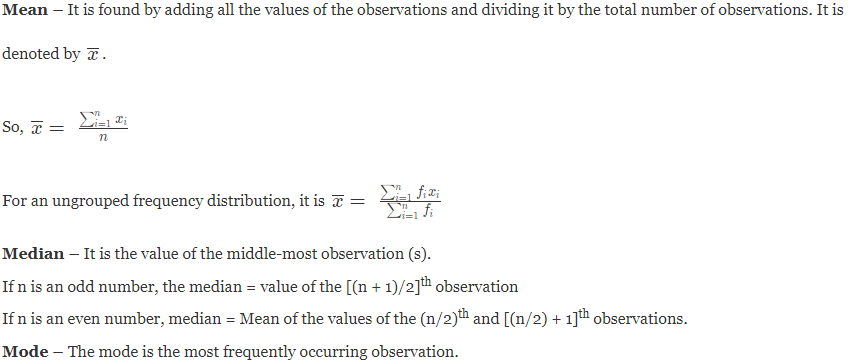Ex. 14.1 – 2 questions (2 short answers)

Ex. 14.2 – 9 questions (9 long answers)

Ex. 14.3 – 9 questions (6 short answers, 3 long answers)

Ex. 14.4 – 6 questions (2 short answers, 4 long answers)

Important Formula of Statistics Chapter 14

There are a total of 3 most important formulae: Mean, Median, Mode.

Probability (Chapter 15)

Probability can be defined as how likely an event can occur. There are 4 short answers, 3 long answers, 3 very long answers in exercise 15.1, a student will have to clear.

To enhance the interest in this topic, the lesson contains questions based on real-life events, throughout the given only one exercise.

The chapter’s main focus is on empirical probability. It is defined as the ratio of the number of outcomes in which a specified event occurs to the total number of trials, based on actual experiment.

Important Formula of Probability Chapter 15

The empirical probability P(E) of an event E happening, is given by

P (E) = Number of trials in which the event happened/ The total number of trials

Study Tips for Class 9 Surface Areas and Volumes

The previous chapter: statistics will also play a major role in this lesson, make sure you have learned them well.

### CBSE Class 9 Maths Unit-wise Marks Weightage

In CBSE 9th standard Mathematics there are a total of 6 units each with several topics and subtopics.

Every single unit has their separate marks weightage that help students to predict the marks they can achieve from the particular lesson.

The detailed Unit-wise marks distribution of CBSE Class 9 Maths is given in below table as well as it tells about the assigned marks for Internal assessment.

 S.No. Unit Name Weightage 1 Number Systems 8 2 Algebra 17 3 Coordinate Geometry 4 4 Geometry 28 5 Mensuration 13 6 Statistics and Probability 10 Total 80 20 marks assigned for internal assessment (I.A).

As per the marking scheme, 80 marks are separated for theory parts whilst students can score 20 marks with the help of Internal Assessment.

### Why Class 9 Maths NCERT Solutions important?

You may be assuming that NCERT Solutions for class 9 Maths are important to do the homework. However, there are 5 subtle reasons why Class 9 Maths NCERT Solutions are important for students.

1. The solutions are prepared by the mathematics experts by maintaining the higher accuracy. Using the same NCERT Solutions allow students to enhance their accuracy.
2. An accurate method to answer a question is given that helps students to understand the concept clearly.
3. It follows the latest CBSE Syllabus so it becomes an important study material to prepare for the exam as exam pattern has been followed in the solutions.
4. NCERT solutions for class 9 maths are prepared by the professional so they have removed the useless things and written the answer of each question precisely.
5. The solutions are helpful to prepare for the annual exam as well as it helps in clearing the scholarship and olympiad exams.

### How to Study NCERT solutions for class 9 maths effectively?

All students should save their time so that they can utilise them in learning and that is why we provide the Class 9 Maths NCERT Solutions that help students to study effectively.

Here’s how you can Study Class 9 Maths NCERT Solutions effectively:-

1. Use NCERT Solutions to Cross check your answer. Doing this helps you to save lots of time from wasting.

2. Learn different methods by using the solutions as it helps you to easily answer the complex questions without feeling devastated.

3. The solution contains the best collection of Important Questions that helps you to learn at the same time prepare for the exam.

### Why is Selfstudys best for NCERT Solutions of Class 9 Maths?

Selfstudys is an online learning platform where subject matter experts develop the NCERT Solutions of class 9 maths as well as for other subjects.

Our solutions are helping students for more than 3 years to prepare for the exam and to ace the board and competitive examination.

Our experts keep in mind the exam pattern and syllabus while preparing the NCERT Solutions. Also, they try their best to make the solutions so simple that students can effectively study them.

Therefore, we believe that Selfstudys is best for NCERT Solutions of Class 9 Maths.

#### Tips to use NCERT Solutions for Class 9 Maths

There is no hard and fast rule to use the NCERT Solutions of Class 9 Maths, but following a systematic approach can help students to study effectively and score higher marks.

Here are some points to keep in mind while referring to the Class 9 Maths NCERT Solutions.

1. It is not advised to the students to copy paste the answers from the NCERT Solutions of 9th mathematics as it will lead them to not learn anything.
2. Ideally, the solutions are prepared to assist students to clear their concepts. Thus, referring to the solution is a great way to revise and practice the most important questions.
3. Sometimes it’s really hard to know what could be the best method of solving a question, but referring to the solution book enables the learners to know how to solve a question easily.

FAQs
Need answers? Find them here...

Yes! You can get the solutions of class 9 maths NCERT. On Selfstudys you can access the NCERT Class 9 Maths Solutions chapter wise for all the exercises. It is free to download in PDF file format.

Since Mathematics is a subject that requires regular practice to become proficient in it, students are suggested to solve the questions from the NCERT Solutions for Class 9 Maths daily. By solving it daily, they can increase their understanding in the subjects along with that their confidence level will be higher.

To perform best in term 1 exam class 9 maths you need to be thorough with all the given chapters and their topics. Along with that, intensive practice for all the exercises are crucial. So, practice the questions and understand all the given concepts. To make that process a bit easier you can refer to the Class 9 Maths NCERT Solutions, Click Here to get.

To learn the Class 9 Maths chapters in a fast and efficient way you should use the NCERT Solutions and practice the questions as much as possible. The NCERT Class 9 Maths Textbook contains numerous exercises and questions. You should have a thorough practice of all those questions in order to learn maths faster and efficiently.

There is no deletion in the exercises available in class 9 maths.

Apart from the Geometry chapter, the 9th maths is not difficult.

By building conceptual knowledge in class 9th maths, a student can score higher marks. However, they need to do lots of practice on the questions.

Like the previous year there is no reduction in class 9th maths syllabus, however, to know the reduction in syllabus of class 9, it is advised to go through the latest syllabus.

From all the 15 chapters in class 9 maths, the Number System chapter 1 is the easiest.×
Open Now